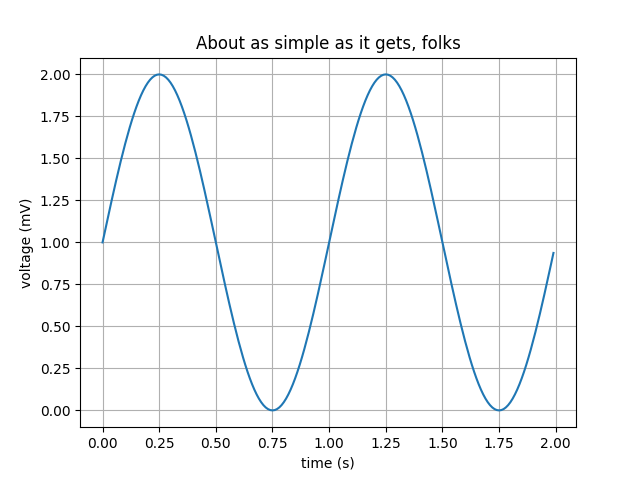You are reading an old version of the documentation (v2.2.3). For the latest version see https://matplotlib.org/stable/gallery/lines_bars_and_markers/simple_plot.html

# Simple Plot¶

Create a simple plot.

```import matplotlib
import matplotlib.pyplot as plt
import numpy as np

# Data for plotting
t = np.arange(0.0, 2.0, 0.01)
s = 1 + np.sin(2 * np.pi * t)

fig, ax = plt.subplots()
ax.plot(t, s)

ax.set(xlabel='time (s)', ylabel='voltage (mV)',
title='About as simple as it gets, folks')
ax.grid()

fig.savefig("test.png")
plt.show()
```